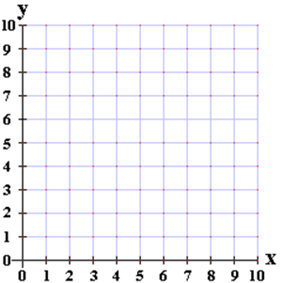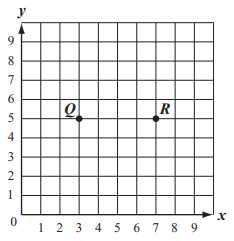# Patterns and the Coordinate Plane

## Objective

Draw shapes on a coordinate plane.

## Common Core Standards

### Core Standards

?

• 5.G.A.1 — Use a pair of perpendicular number lines, called axes, to define a coordinate system, with the intersection of the lines (the origin) arranged to coincide with the 0 on each line and a given point in the plane located by using an ordered pair of numbers, called its coordinates. Understand that the first number indicates how far to travel from the origin in the direction of one axis, and the second number indicates how far to travel in the direction of the second axis, with the convention that the names of the two axes and the coordinates correspond (e.g., x-axis and x-coordinate, y-axis and y-coordinate).

• 5.G.A.2 — Represent real world and mathematical problems by graphing points in the first quadrant of the coordinate plane, and interpret coordinate values of points in the context of the situation.

?

• 4.G.A.2

• 5.G.B.4

## Criteria for Success

?

1. Given a set of points, plot them in the coordinate grid and identify and explain what shape they form when connected (MP.3).
2. Construct a specific shape on the coordinate grid given some or none of its points.

## Tips for Teachers

?

In addition to or in place of the Problem Set, you could have students play a modified version of Battleship. Instead of students’ targets being a straight line of points, make the targets various shapes (e.g., a rectangle, a trapezoid, a right triangle, etc.). Provide students with images of the shapes they need to plot so that they know exactly the dimensions of each to avoid confusion.

#### Fishtank Plus

• Problem Set
• Student Handout Editor
• Vocabulary Package

?

### Problem 1

Using the coordinate grid below:

1. Plot a point A at (1, 1).
2. Plot a point B at (6, 1).
3. Plot a point C at (3, 4).
4. Plot a point D at (8, 4).
5. Connect the points in order, then connect point D back to point A
6. Name all the ways you can classify the shape you have formed.#### References

Achievement First Grade 5, Unit 9, Lesson 4 (2017-2018)Independent Practice, Bachelor Level #1

Grade 5, Unit 9, Lesson 4 (2017-2018) is made available by Achievement First as a part of their Open Source web portal under a CC BY 4.0 license. Copyright © 1999-2017 Achievement First. Accessed May 25, 2018, 2:04 p.m..

Modified by The Match Foundation, Inc.

### Problem 2

Alonso wants to create a triangle on the coordinate grid below. Two of the vertices are located at point (2, 3) and point (7, 3).1. If Alonso wants to make a right triangle, what could be the ordered pair of the third vertex?
2. Alonso decides to use his original two points to form a square instead.  Where should he place the other two points, and why?

#### References

Grade 5, Unit 9, Lesson 4 (2017-2018) is made available by Achievement First as a part of their Open Source web portal under a CC BY 4.0 license. Copyright © 1999-2017 Achievement First. Accessed May 25, 2018, 2:04 p.m..

Modified by The Match Foundation, Inc.

## Problem Set & Homework

#### Discussion of Problem Set

• What other words can you use to describe the shape in #1? How can the shape be classified as all of those words simultaneously? What about the shape in #2(a)?
• How did you create the shape in #2(b)?
• How did you decide where to plot the third vertex to create an obtuse triangle in #3? How did you determine which point would create an acute triangle in #4?
• How did you solve #8? What made this task more difficult than the previous ones?

?

Jillian plotted points Q and R on a coordinate grid, as shown below.Jillian wants to plot point S so that when points QR, and S are connected they form the vertices of a right triangle. Write an ordered pair that represents where Jillian should plot point S.

#### References

Massachusetts Department of Elementary and Secondary Education Spring 2014 Grade 5 Mathematics TestQuestion #17

Spring 2014 Grade 5 Mathematics Test is made available by the Massachusetts Department of Elementary and Secondary Education. © 2017 Commonwealth of Massachusetts. Accessed Feb. 5, 2018, 12:12 p.m..

?